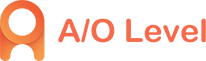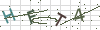• 4008506500
• 1930985500
• peixunmm1
• Suite 601, Level 6, 109 Pitt Street, Sydney,NSW 2000

# 小试牛刀，A-Level数学考试真题练习题

2019-09-12 10:59:51 1488人已读 分享到

对于A-Level课程中的数学考试，很多同学都有点不以为然，大多数考查的知识初中高中都学过了，所以大多数同学都觉得A-Level数学已经是囊中之物了，不需要备考，那么真的是这样吗？你的数学水平如何，不妨做几道真题来证明一下！本文就为大家分享几道A-Level数学考试真题，做做看吧！

1.若关于x的方程a(x—1)?=2x2—2是一元二次方程，则a的值是( )

A.2

B.—2

C.0

D.不等于21. If the equation a(x-1)_=2x 2-2 about X is a quadratic equation of one variable, then the value of a is (）

A.2

B. - 2

C.0

D. is not equal to 2.

2.若x=1是方程ax2+bx+c=0的解，则( )

A.a+b+c=1

B. a—b+c=0

C.a+b+c=0

D.a—b—c=0

2. If x = 1 is the solution of equation AX2 + BX + C = 0, then ()

A.a+b+c=1

B. a - b+c=0

C.a+b+c=0

D.a-b-c=0

3.关于x2=—2的说法，正确的是( )

A.由于x2≥0，故x2不可能等于—2，因此这不是一个方程

B.x2=—2是一个方程，但它没有一次项，因此不是一元二次方程

C.x2=—2是一个一元二次方程

D.x2=—2是一个一元二次方程，但不能解

3. The correct statement about x2=-2 is ()

A. Because x 2 is greater than or equal to 0, x 2 cannot be equal to - 2, so this is not an equation.

B.x2=-2 is an equation, but it has no first term, so it is not a quadratic equation of one variable.

C.x2=-2 is a quadratic equation of one variable.

D.x2=-2 is a quadratic equation of one variable, but it can not be solved.

怎么样？上面的几道A-Level数学考试选择题你都答对了吗？本文关于A-Level数学考试真题练习的内容就是这样了，希望能够对考生们有所帮助，如果还想了解更多alevel考试大纲、alevel考试题等方面的问题，可咨询我们的客服。• ### A-level资讯课堂：英国留学的预科和A-Level课程有哪些区别4008506500